# Gated2Gated: Self-Supervised Depth Estimation from Gated Images

• Amanpreet Walia

• Stefanie Walz

• Mario Bijelic

• Fahim Mannan

• Frank Julca-Aguilar

• Michael Langer

• Werner Ritter

• Felix Heide

CVPR 2022 (Oral)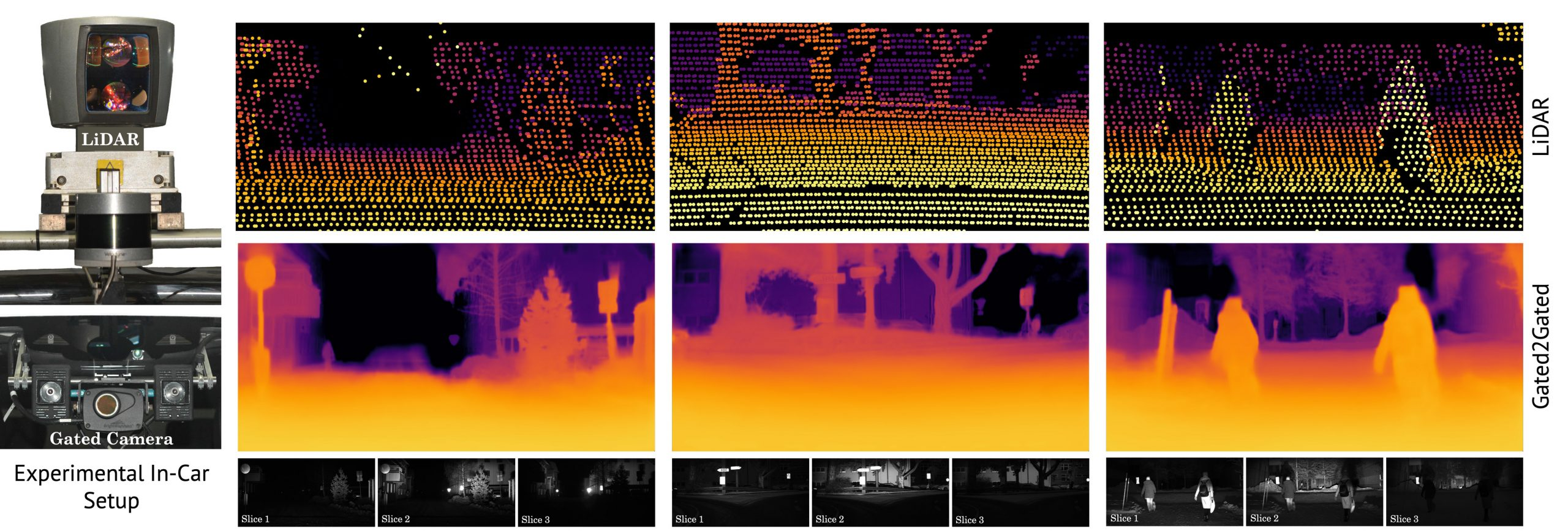An example gated imaging system is pictured in the bottom left and consists of a synchronized camera and a not shown VECSL flash illumination source. The system allows to integrate the scene response for narrow depth ranges as illustrated in the bottom row. Therefore, the overlapping gated slices contain implicit depth information according to the time-of-flight principle at image resolution. In comparison the illustrated LiDAR sensors in the top left send out point wise illumination pulses causing a sparse depth representation depicted in the top row. Our proposed self-supervised Gated2Gated learning technique recovers this dense depth information (middle row) from the shown set of three gated images, by learning from temporal and gated illumination cues.

Gated cameras hold promise as an alternative to scanning LiDAR sensors with high-resolution 3D depth that is robust to back-scatter in fog, snow, and rain. Instead of sequentially scanning a scene and directly recording depth via the photon time-of-flight, as in pulsed LiDAR sensors, gated imagers encode depth in the relative intensity of a handful of gated slices, captured at megapixel resolution. Although existing methods have shown that it is possible to decode high-resolution depth from such measurements, these methods require synchronized and calibrated LiDAR to supervise the gated depth decoder - prohibiting fast adoption across geographies, training on large unpaired datasets, and exploring alternative applications outside of automotive use cases. In this work, we fill this gap and propose an entirely self-supervised depth estimation method that uses gated intensity profiles and temporal consistency as a training signal. The proposed model is trained end-to-end from gated video sequences, does not require LiDAR or RGB data, and learns to estimate absolute depth values. We take gated slices as input and disentangle the estimation of the scene albedo, depth, and ambient light, which are then used to learn to reconstruct the input slices through a cyclic loss. We rely on temporal consistency between a given frame and neighboring gated slices to estimate depth in regions with shadows and reflections. We experimentally validate that the proposed approach outperforms existing supervised and self-supervised depth estimation methods based on monocular RGB and stereo images, and even supervised methods based on gated images.

## Paper

Gated2Gated: Self-Supervised Depth Estimation from Gated Images

Amanpreet Walia, Stefanie Walz, Mario Bijelic, Fahim Mannan, Frank Julca-Aguilar, Michael Langer, Werner Ritter, Felix Heide

CVPR 2022 (Oral)

## Experimental Results in Diverse Driving Conditions

In the following qualitative results of the proposed selft-supervised depth estimation method Gated2Gated are compared to existing methods that use gated images (Gated2Depth), lidar (Sparse2Dense) or RGB camera (PackNet)  as input.  Gated2Depth uses the same three gated slices as Gated2Gated, but is supervised by LiDAR and simulation data during training. Sparse2Dense uses sparse LiDAR data and a monocular RGB image as input and upsamples the LiDAR depth with monocular texture cues. Lastly, PackNet is the next best self-supervised monocular depth estimation method with RGB data as input.

##### Measurement Decomposition

For the self-supervision and the loop closer reconstructing the gated slices from the depth cues the proposed method also has to learn to predict the albedo and ambient illumination of the scene. The albedo represents a joint state of illumination and  reflectivity while the ambient image captures the surrounding sunlight and active light sources, such as vehicle lights or traffic lights.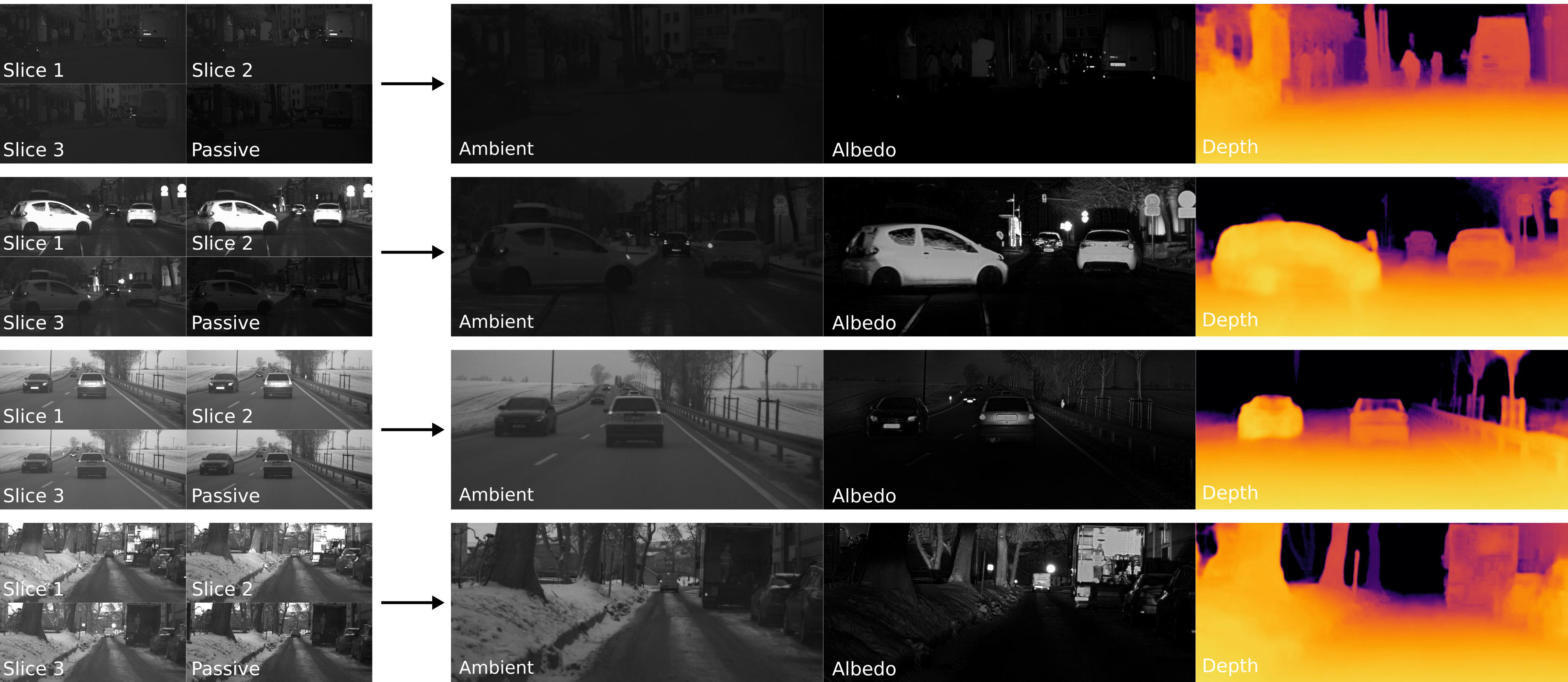### Self-Supervised Gated Depth Estimation

To achieve the results shown above, we propose a self-supervised depth estimation method for gated images that learns to predict depth $\mathbf{\hat{r}}$ without ground truth supervision from LiDAR or simulation. To this end, we rely on cyclic measurement consistency of gated images and temporal consistency in the depth predictions itself. Self-supervision allows us to overcome the limited depth range $80$ m of methods trained on LiDAR ground-truth and removes complex synchronization processes between LiDAR and cameras. Furthermore, we can train our models on harsh weather conditions, e.g., fog, rain, or snow, where LiDAR-based ground-truth fails. The proposed architecture is composed of the following three networks

• The first network predicts a dense depth map per gated tensor $\mathbf{Z}_t$, denoted as $f_{\mathbf{r}}: \mathbf{Z}_t \to \mathbf{\hat{r}}$.
• The second network takes the gated images $\mathbf{Z}_t$ as input, and predicts ambient and albedo, denoted as $f_{\mathbf{\Lambda\alpha}}: \mathbf{Z}_t \to (\mathbf{\hat{\boldsymbol{\Lambda}}}_t, \mathbf{\hat{\boldsymbol{\alpha}}}_t)$.
• The third network takes two temporally adjacent gated tensors as input $(\mathbf{Z}_t, \mathbf{Z}_n)$, and predicts a rigid 6 DoF pose transformation $\mathbf{\hat{\boldsymbol{X}}}$ from $\mathbf{Z}_n$ to $\mathbf{Z}_t$.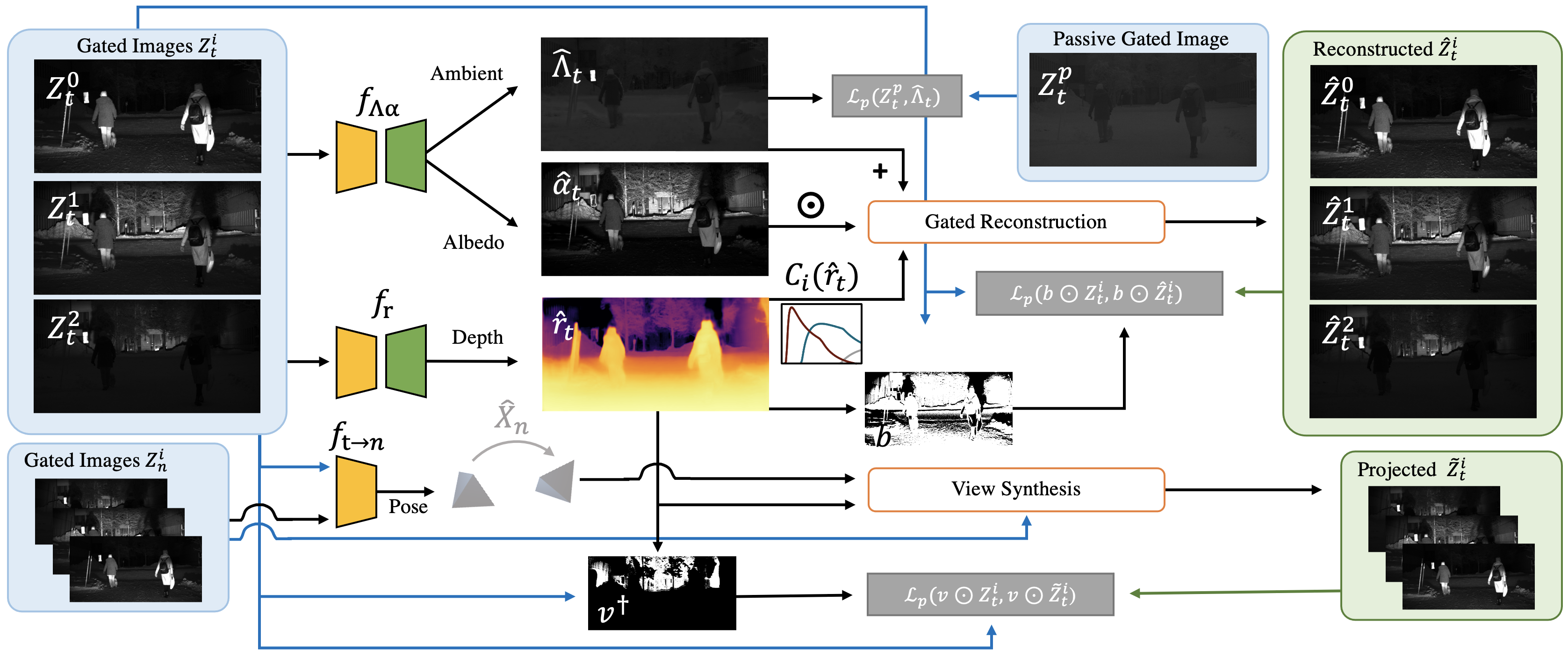### Cyclic Gated Consistency

The propoosed cyclic gated consistency loss supervises the predicted depth $\mathbf{\hat{r}}_t$, ambient $\mathbf{\hat{\boldsymbol{\Lambda}}}_t$ and albedo $\mathbf{\hat{\boldsymbol{\alpha}}}_t$, by reconstructing the gated slices. The final model then can be written as:

$$\hat{\mathbf{Z}}_t^i = \mathbf{\hat{\boldsymbol{\alpha}}}_t C_i(\mathbf{\hat{r}}_t) + \mathbf{\hat{\boldsymbol{\Lambda}}}_t$$

The gated slices $\hat{\mathbf{Z}}_t^i$ can be reconstructed using the proposed cyclic gated consistency, which enforces a match between a predicted depth estimate and the ground truth $\hat{\mathbf{Z}}^i_t$ measurement it came from. However, comparison between the ground-truth and the reconstructon can fail in practice as a result of severe multipath effects, missing illumination due to occlusion, and saturation for retro-reflective signs. To tackle these issues, we introduce the cycle loss mask $b$ that optimize the performance of the cyclic self-supervised model in such conditions.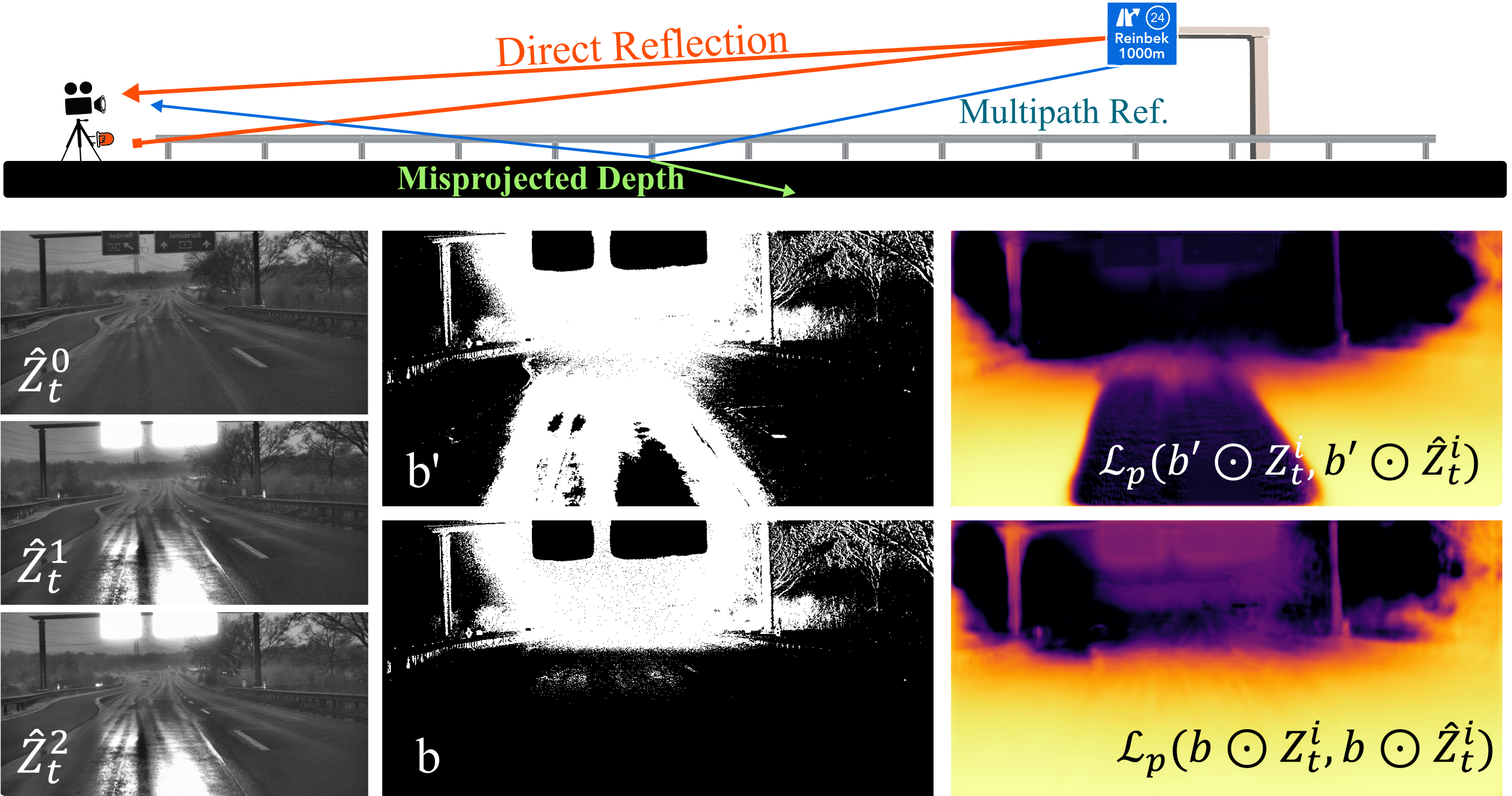### Temporal Consistency

We rely on view synthesis to introduce temporal consistency between adjacent gated images during training. Specifically, we reconstruct the view of the central gated image $\hat{\mathbf{Z}}_t$ inbetween its temporal neighbors $\mathbf{Z}_n$ using the camera matrix $K$, the predicted depth $\mathbf{\hat{r}}_t$ and camera pose transformation $\hat{\mathbf{X}}_{t\rightarrow n}$. To handle the dynamic scene objects, we use gated illumination cues to construct infinity correction masks $v$ to prevent projections to infinity. These masks allow us to filter mispredicted depth values stemming from moving objects which can not be captured by the rigid body transformation $\hat{\mathbf{X}}_{t\rightarrow n}$.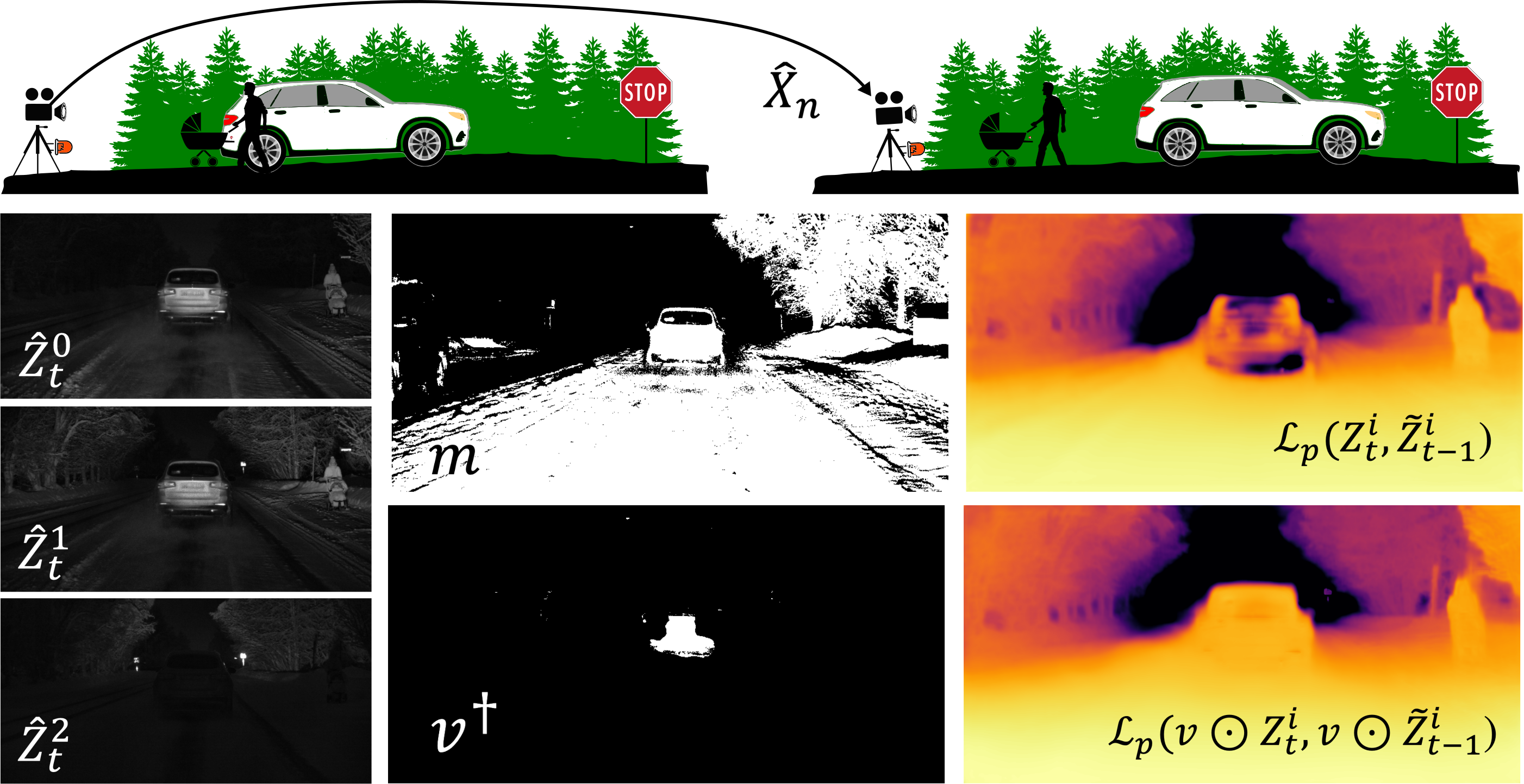## Related Publications

 Tobias Gruber, Frank D. Julca-Aguilar, Mario Bijelic, Werner Ritter, Klaus Dietmayer, and Felix Heide. Gated2depth: Real-time dense lidar from gated images. The IEEE International Conference on Computer Vision, 2019.

 Mario Bijelic, Tobias Gruber, Fahim Mannan, Florian Kraus, Werner Ritter, Klaus Dietmayer, and Felix Heide. Seeing Through Fog Without Seeing Fog: Deep Multimodal Sensor Fusion in Unseen Adverse Weather. The IEEE Conference on Computer Vision and Pattern Recognition (CVPR), 2020.

 Frank Julca-Aguilar, Jason Taylor, Mario Bijelic, Fahim Mannan, Ethan Tseng, and Felix Heide. Gated3D: Monocular 3D Object Detection From Temporal Illumination Cues. The IEEE International Conference on Computer Vision, 2021.

 V. Guizilini,, R. Ambrus, S. Pillai, A. Raventos and  A. Gaidon. 3d packing for self-supervised monocular depth estimation. The IEEE International Conference on Computer Vision, 2020.

 Fangchang Ma and Sertac Karaman. Sparse-to-dense: Depth prediction from sparse depth samples and a single image. The IEEE international conference on robotics and automation (ICRA), 2018.